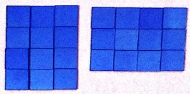Level: Year 2 and Year 3

Author: Anna Bock

Description:

Multiplication facts can be represented as an array.  An array is a rectangular (including square) arrangement of objects, counters or tiles in equal rows. To help children describe an array we need to focus on ‘How many rows?’ ‘How many in each row?’, this will support them when representing an array in numbers. The purpose of this game is to provide the opportunity for students to construct arrays and to describe them in words and numbers. The game reinforces the array model of multiplication. This game can be played many times and will support students to gain confidence in using the array model for multiplication and to discover that the order in which two numbers are multiplied does not affect the product, this is the commutative property/law.

THE ARRAY GAME

Materials: Counters, two different coloured dice, pencils and paper

Players:  2

Objective: To create a variety of arraysInstructions:

• Roll both the dice
• One dice is to represent the number of rows
• The other dice represents how many in each row
• Make the array using the counters
• Describe the array made e.g. 3 rows of 4 or 4 rows of 3
• Draw the array that has been made and describe it in words or numbers

We want students to:

• Make a variety of arrays
• Explain and describe the array they have made
• Draw the array they have made
• Describe their array in words and/or numbers
• See the link that 3 rows of 4 is also 4 rows of 3 when it is turned around, this is the commutative law

Notes for parents: Multiplication facts can be represented as an array.  This game can be played many times and it will support your child to gain confidence in using the array model for multiplication. Encourage your child to describe their array in words for example ‘four rows and three in each row’, or in numbers as 4×3

This activity covers the following Australian Curriculum – Mathematics Content:

Year 2:

• Recognise and represent multiplication as repeated addition, groups and arrays (ACMNA031)

Year 3:

• Recall multiplication facts of two, three, five and ten and related division facts (ACMNA056)
• Represent and solve problems involving multiplication using efficient mental and written strategies and appropriate digital technologies (ACMNA057)

Modified from © Ann Downton for CTLM Intake 4 P-2 Program, Mathematics Teaching and Learning Research Centre (ACU) and Catholic Education Office (Melbourne) 2011 (Day 5, October 2011)